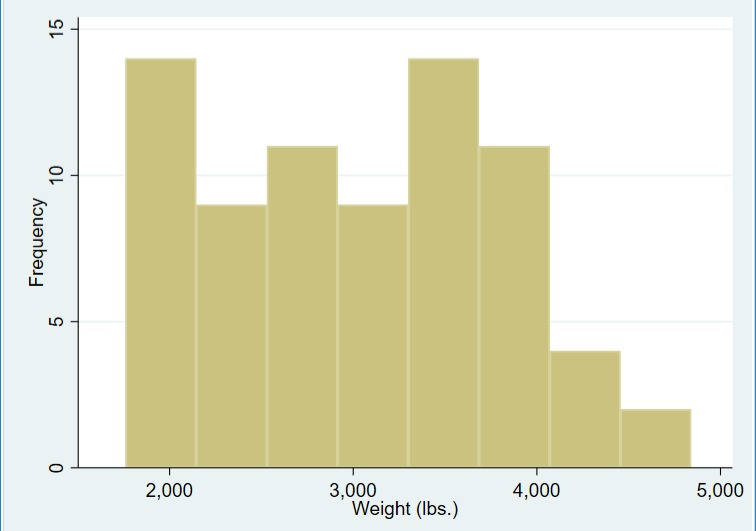Search

# Categorical Histograms

A histogram can be used to show either continuous or categorical data in a bar graph. For continuous data the histogram command in Stata will put the data into artificial categories called bins. For example, if you have a list of heights for 1000 people and you run the histogram command on that data, it will organize the heights into ranges. Each range is shown as a bar along the x-axis, and each bar represents the number of people whose height falls into that range. In this way the histogram command has created height categories to give a visual understanding of the distribution of heights in the data.

If the data you have is categorical then sometimes it will require some modification before a histogram can be generated. This is because each category must be represented as a number in order to generate a histogram from the variable. You cannot generate a histogram from a string variable.

If the categorical variable you have is represented as a string you can use the encode command, which provides a simple way of converting strings to a numeric format so you can generate a histrogram. The encode command works by assigning a number to each different string in the variable, starting at 1.

Note: If possible try to make sure your string categories use the same capitalization and that all the characters are the same. If your categories are not consistent as strings you can end up with a category represented by more than one number which will skew the results of the histogram.

For more information about the encode command and how it works, check out this Tech Tip: Encode and Decode Commands.

## How to Use:

For continuous variables:

For categorical string variables:

## Worked Example 1:

In this example I use the auto dataset to generate a histogram using the car weight data. In the command pane I type the following:

This produces the following output:This is a simple histogram that represents the distribution of car weights in the auto dataset. Looking at the y-axis you’ll notice that the distribution is shown in terms of density. In this case density is not as helpful an indicator of distribution as frequency, so I am going to generate a new histogram that shows the distribution using frequency instead. In the command pane I type the following:

Which produces the following graph:In this histogram the y-axis is telling us that each bar represents approximately 10-15 cars, until the last two bars which contain less than 5 cars. This is a more tangible representation of car weight distribution than the density shown in the previous histogram.

## Worked Example 2:

In this example I am going to create a histogram showing the different makes of car as different categories. This requires some modification of the “make” variable before I can generate the histogram. First I need to isolate the make of the car and generate a new variable containing only the make, as the “make” variable in the auto dataset contains both the make and model as a single string. To do this I use the split command. I can then encode my new “make1” variable containing only the make. Once encoded I can then generate a histogram of the distribution of car makers in the dataset. In the command pane I type the following:

This gives me the following histogram:This histogram shows each make as a number along the x-axis. There are 23 different car makes in this dataset. In order to show each car maker represented on the x-axis you need to attach the labels for each number to the histogram. To do this I type the following in the command pane:

This code is asking that Stata show all 23 different car makes as separate bars on the histogram, which is what the option bin(23) refers to. The frequency option is used so the y-axis will show as frequency instead of density. Finally the xlabel(#15, valuelabel) is specifying that the labels be shown as the text of each car make rather than each car make’s numeric identifier.

This produces the following histogram:As there are 23 car makes in the histogram they are not all able to be shown in text, as the labels would become too crowded and unreadable. You can produce a histogram showing the numbers instead of the text, but you would have to identify what car make each number represents in the histogram’s accompanying text.